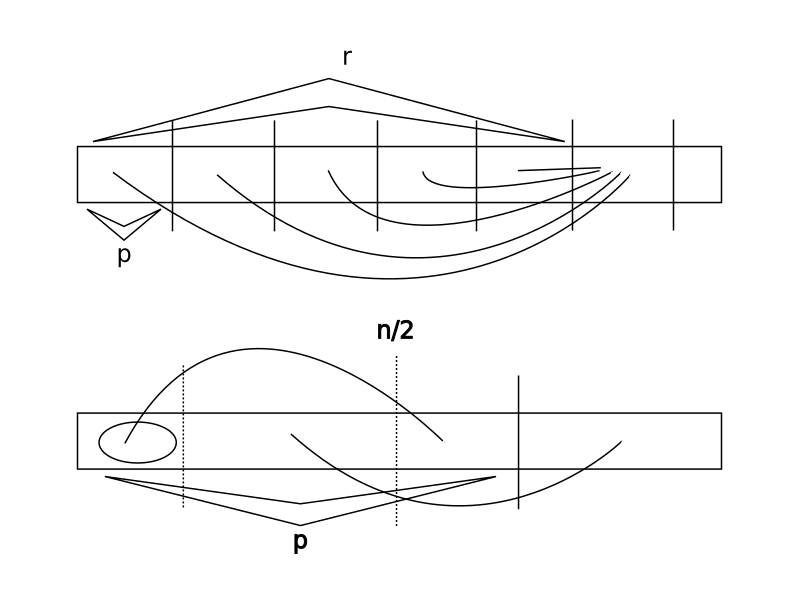# 字符串的期望本质不同子串个数

$$E_w(x)=\frac{x^{|w|}}{(1-mx)(x^{|w|}+(1-mx)c_w(x))}$$

$$f_v=S_v-\sum_{j|i\subset j}f_j$$

\begin{aligned} F_1(x)&=x\\ F_2(x)&=-x+3x^3\\ F_3(x)&=-3x^2+6x^3 \end{aligned}

• $[x^0]F_n(x)=0$：这个很显然，如果你字符集大小为 $0$ 根本无法组成任何字符串。
• $[x^n]F_n(x)=\frac{n(n+1)}2$。这个是尚未证明的猜想。

$\Xi(v)$ 为真，当且仅当 $v$ 为空集；或 $v_0=1$，且满足下面两种互不相交的条件之一：

• $\pi(v)\le\left\lfloor\frac{|v|}2\right\rfloor$，且对于任意 $\pi(v)$ 的倍数 $k$（$k<|v|$）都有 $v_k=1$；

且，令 $r=\pi(v)(\left\lfloor\frac n{\pi(v)}\right\rfloor-1)$、$w=v_{r\dots(|v|-1)}$ 时，有 $\pi(w)\ge p$ 或 $\pi(w)>(n-r-\pi(v))+(\pi(v),\pi(w))$；

且 $\Xi(w)$ 为真。

• $\pi(v)>\left\lfloor\frac{|v|}2\right\rfloor$，且 $\Xi(v_{r\dots(|v|-1)})$ 为真。

• $\pi(v)\le\left\lfloor\frac{|v|}2\right\rfloor$。我们首先检查 $q$ 中的元素是否和 $\pi(v)$ 冲突（即，是否存在 $i$ 使得 $p\nmid i$ 且 $q_i=1$），然后将所有 $p$ 的所有小于 $|v|$ 的倍数 $i$ 标记 $q_i=1$，接着递归到 $n’=n-r$ 继续进行枚举。
• $\pi(v)>\left\lfloor\frac{|v|}2\right\rfloor$ 且 $\pi(v)\ne+\infty$。我们直接递归到 $n’=n-\pi(v)$ 进行枚举。
• $\pi(v)=+\infty$。这种情况我们已经找到了一种合法的 $v$，将其输出并返回。• $\pi(v)\le\left\lfloor\frac{|v|}2\right\rfloor$（图一）。此时整个字符串的自由元数目等价于剩下那一段的自由元数目，不需要额外添加。
• $\pi(v)>\left\lfloor\frac{|v|}2\right\rfloor$（图二）。自由元数目等价于圈起来的一部分加上后面剩下一部分的自由元。
• $\pi(v)=+\infty$。自由元数目为 $0$。

• $insert(x,v)$：插入二元组 $(x,v)$，其中 $x$ 为一个集合（集合元素值域即为 $[1,n]$），$v$ 为整数。

• $query(y)$：统计所有满足 $x\supseteq y$ 的二元组 $(x,v)$ 中 $v$ 的和。

Mivik

2021-01-26

2023-02-22